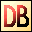DelphiBasicsRadToDegFunction Converts a radian value to degrees Math unit
 function RadToDeg ( const Radians : Extended ) : Extended;
Description
The RadToDeg function is a mathematical function converting the Radians value into Degrees.

PI radians = 180 degrees
Related commands
 CelsiusToFahrenheit Convert a celsius temperature into fahrenheit Convert Convert one measurement value to another DegToRad Convert a degrees value to radians FahrenheitToCelsius Convert a fahrenheit temperature into celsius
Author links

Buy Website Traffic at
Buywebsitetrafficexperts.com

Buy Proxies at
Buyproxies.ioDownload this web site as a Windows program.

 Example code : Use to display the result of an ArcSin calculation in degrees // Full Unit code.// -----------------------------------------------------------// You must store this code in a unit called Unit1 with a form// called Form1 that has an OnCreate event called FormCreate. unit Unit1; interface uses  Math,   // Unit containing the RadToDeg command  SysUtils,  Forms, Dialogs; type  TForm1 = class(TForm)    procedure FormCreate(Sender: TObject);  end; var  Form1: TForm1; implementation{\$R *.dfm} // Include form definitions procedure TForm1.FormCreate(Sender: TObject); var   float : single; begin  // The ArcSin of 0.5 should give 30 degrees   float := ArcSin(0.5);  float := RadToDeg(float);  // Convert result to degrees   ShowMessage('ArcSin of 0.5 = '+FloatToStr(float)+' degrees'); end; end. Hide full unit code ArcSin of 0.5 = 30 degrees
 Delphi Programming © Neil Moffatt 2002 - 2018. All rights reserved.  |  Home Page